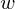Here we come to answer the question: Why should we use Linear regression? What are its strengths over the other algorithms?

Even being one of the most simple predictive methods in Machine learning, Linear regression is still frequently used in practice, and here are the 7 reasons:

Simplicity

Yes, it is simple.

Unlike Support Vector Machine with complicated maths, Deep learning with many mysteries that humans have yet to understand, Linear regression is very simple and straightforward.

Computing a Linear model is also very fast. Hence, it is usually used as a prototype model, giving a baseline for benchmarking other expensive algorithms.

Transparency and High-interpretability

Linear regression is easy to interpret. That means looking at the model, we can understand why it gives this output given this sample. This property is very important when we need to make a big decision because we don’t want to blindly follow a black-box model that we don’t know anything about it at all.

Linear regression also gives us a sense of feature importance. This information can affect our perspective of the problem, as well as pointing out the direction we should focus on to get more out of the data.

Feature selection

Regularization (especially Lasso) helps eliminate redundant features.

Linear regression is often used as a first-step model, whose main role is to remove unwanted features from a bag that has many. By eliminating those features, other models will be fitted faster, and less prone to capture the noise instead of underlying trends.

Determinism

When you choose to use a convex cost function (for example, Mean-Squared-Error), the Linear Regressor can be computed deterministically.

Determinism means that we are guaranteed to get the best set-of-weights () given the input data. This property is not always true for predictive models. Algorithms like Decision Tree or Deep learning are stochastic, which means they have some randomization inside, which may lead to different results each time we train the model, and the trained model is not likely the global optimum.

Reliability in practice

Despite being so simple, Linear regression is functioning quite well in most practical problems. Another algorithm with this property is Naive Bayes. Both of them, although requiring strong assumptions, can still work in the cases when some of the assumptions are violated.

Note that Linear regression’s performance in those cases is just moderately good, not the best.

Ability to estimate the Confidence Intervals

We can compute the Confidence intervals for Regressor coefficients. This helps with estimating the true value of the coefficients – the representative of how strong the relationship of each predictor with the response variable.

Online-learning is supported

With the Gradient Descent method for fitting the set of parameters, Linear Regression supports learning online, a property that modern Machine Learning applications highly appreciate.

You can find the full series of blogs on Linear regression here.